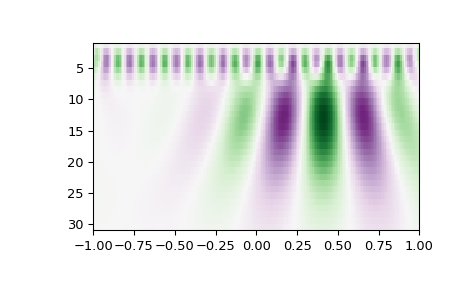# scipy.signal.cwt¶

scipy.signal.cwt(data, wavelet, widths)[source]

Continuous wavelet transform.

Performs a continuous wavelet transform on data, using the wavelet function. A CWT performs a convolution with data using the wavelet function, which is characterized by a width parameter and length parameter.

Parameters: data : (N,) ndarray data on which to perform the transform. wavelet : function Wavelet function, which should take 2 arguments. The first argument is the number of points that the returned vector will have (len(wavelet(length,width)) == length). The second is a width parameter, defining the size of the wavelet (e.g. standard deviation of a gaussian). See ricker, which satisfies these requirements. widths : (M,) sequence Widths to use for transform. cwt: (M, N) ndarray Will have shape of (len(widths), len(data)).

Notes

length = min(10 * width[ii], len(data))
cwt[ii,:] = signal.convolve(data, wavelet(length,
width[ii]), mode='same')


Examples

>>> from scipy import signal
>>> import matplotlib.pyplot as plt
>>> t = np.linspace(-1, 1, 200, endpoint=False)
>>> sig  = np.cos(2 * np.pi * 7 * t) + signal.gausspulse(t - 0.4, fc=2)
>>> widths = np.arange(1, 31)
>>> cwtmatr = signal.cwt(sig, signal.ricker, widths)
>>> plt.imshow(cwtmatr, extent=[-1, 1, 31, 1], cmap='PRGn', aspect='auto',
...            vmax=abs(cwtmatr).max(), vmin=-abs(cwtmatr).max())
>>> plt.show()#### Previous topic

scipy.signal.ricker

#### Next topic

scipy.signal.find_peaks_cwt